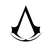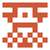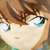# 3544. 小迷妹在哪儿bool cmp(const data &aa,const data &bb) {
return aa.a * bb.t > aa.t * bb.a;
}(t-t1)a1+(t-t1-t2)a2=(t-t2)a2+(t-t1-t2)a1;化简得到：a1t1=a2t1,发现区分迷妹价值和时间t无关，于是你可以随便设一个t=300，然后比较val_1=(t-t1)a1+(t-t1-t2)a2和val_2=(t-t2)a2+(t-t1-t2)a1哪个大，大的那个说明先找的那个迷妹性价比高。